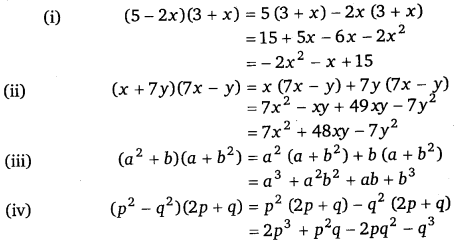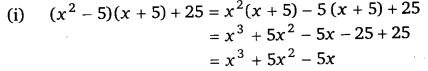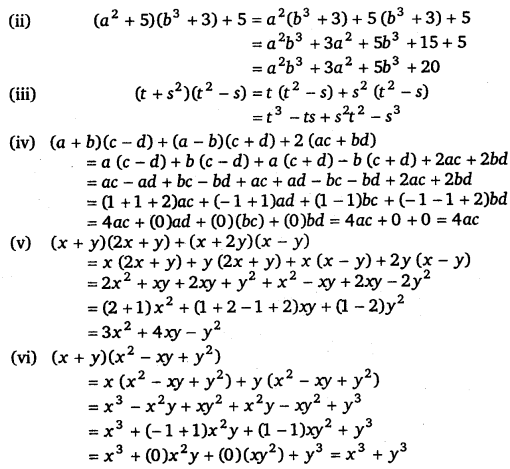# Class 8 Maths NCERT Solutions for Chapter – 9 Algebraic Expressions and Identities Ex – 9.4

### Algebraic Expressions and Identities

Question 1.
Multiply the binomials :
(i)
(2x + 5) and (4x – 3)
(ii) (y – 8) and (3y – 4)
(iii) (2.5l – 0.5m) and (2.5l + 0.5m)
(iv) (a + 3b) and (x + 5)
(v) (2pq + 3q2) and (3pq – 2q2)
(vi)$\left( \frac { 3 }{ 4 } { a }^{ 2 }+3{ b }^{ 2 } \right) and\quad 4\left( { a }^{ 2 }-\frac { 2 }{ 3 } { b }^{ 2 } \right)$

Solution:Question 2.
Find the product :
(i)
(5 – 2x)(3 + x)
(ii) (x + 7y)(7x – y)
(iii) (a2 + b)(a + b2 )
(iv) (p2 – q2)(2p + q)

Solution:Ex 9.4 Class 8 Maths Question 3.
Simplify :
(i) (x2 – 5)(x + 5) + 25
(ii) (a2 +5)(b3 +3)+ 5
(iii) (t +s2)(2 – s)
(iv) (a + b)(c – d) + (a – b)(c + d) + 2 (ac + bd)
(v) (x + y)(2x + y)+ (x + 2y)(x – y)
(vi) (x + y)(x2 – xy + y2)
(vii) (1.5x – 4y) (1.5x + 4y + 3) – 4.5x + 12y
(viii) (a + b + c)(a + b – c)
Solution:#### Class 8 Maths NCERT Solutions for Chapter – 9 Algebraic Expressions and Identities Ex – 9.5

Lorem ipsum dolor sit amet, consectetur adipiscing elit. Phasellus cursus rutrum est nec suscipit. Ut et ultrices nisi. Vivamus id nisl ligula. Nulla sed iaculis ipsum.

Company Name